VIII.D.3 Estimation of Parameters

# IRAS Explanatory Supplement VIII. Sky Coverage, Confusion, Completeness and Reliability D. Point Source Catalog Reliability and Completeness

## D.3 Estimation of Parameters

Chapter Contents | Authors | References

The only data available for estimating the parameters are the observed densities n(N,M) of sources on the sky, observed N out of M possible times. There is no a priori information as to whether these sources are real or false. Let E<x> denote the expectation value of x. Then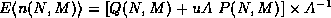(VIII.D.8)

where Q(N,M) and P(N,M) are given respectively by Eqs. (VIII.D.l) and (VIII.D.5) and contain the parameters p and q. It is clear that estimates û, p^ and  q^  of u, p and q can be obtained by replacing the expectation value of n(N,M) in Eq. (VIII.D.8) by the observed values, bearing in mind that the value of n(0,M) is unobservable. The following equations, from which û, p^  and q^  can be estimated, are derived using Eqs. (VIII.D.1, VIII.D5 and VIII.D.8)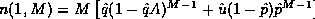(VIII.D.9)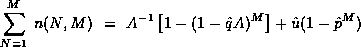(VIII.D.10)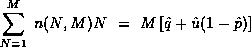(VIII.D.11)

Since (qA) « 1 in the case of this survey, Eq. (VIII.D.9) and (VIII.D.10) can be approximated by: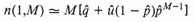(VIII.D.12)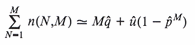(VIII.D.13)

The equations can be greatly simplified for sources of high signal-to-noise ratio when p « 1 if it is assumed that the true source density is not greatly in excess of the false density. Equation (VIII.D.12) becomes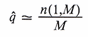(VIII.D.14)

Equations (VIII.D.13) and (VIII.D.14) give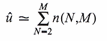(VIII.D.15)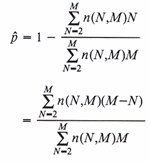(VIII.D.16)

Each of these equations, which are valid only for bright sources, has a simple interpretation. Equation (VIII.D.14) says that the probability of generating false sources is given by the density of single HCONs Pided by the number of chances of seeing them. The contribution of true sources which have lost all but one HCON is neglected. Equation (VIII.D.15) says that all sources with two or more HCONs are real. Finally, Eq. (VIII.D.16) says that the probability of missing an HCON is the sum of all the missed HCONs divided by the total possible number of HCONs; again the contribution of true sources which have lost M - 1 HCONs is neglected.

The parameters u, p and q were estimated using Eqs. (VIII.D.11, 12 and 13). Only preliminary results are presented here because these parameters were directly affected by the last processing steps (clean-up, flux analysis, and high source density processing) used to generate the catalog.

Chapter Contents | Authors | References